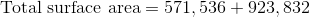## Example Questions

### Example Question #1 : How To Find The Surface Area Of A Pyramid

The Pyramid of Giza has a height of 480 feet. If the length of each side of the base is approximately 756 feet, what is its total surface area? Round to the nearest tenth.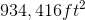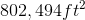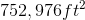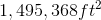Explanation:

If the length of one side is 756 ft, then multiply to find the area of the base.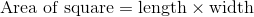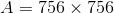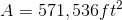Once you've found the area of the base, use the height of the pyramid and half of the side length of the base to determine the length of the side from the apex to the ground using the Pythagorean Theorem.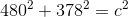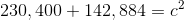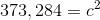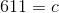Using the side length of the base and the height of each of the triangles that form the pyramid, calculate the area of each triangle, then multiply by 4.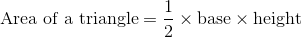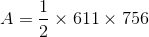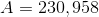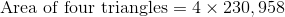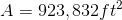Add the surface area of the base to the surface area of the four triangles.